# Graph Worksheet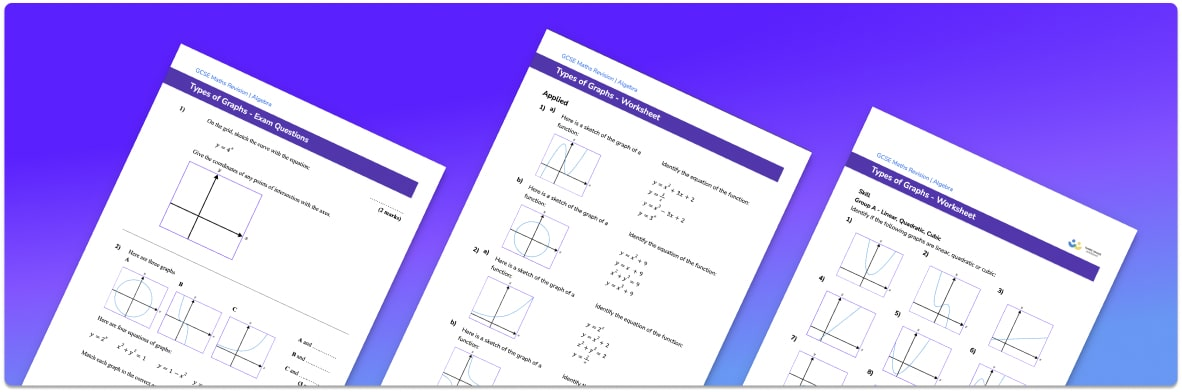• Section 1 of the graph worksheet contains 20+ skills-based graph questions, in 3 groups to support differentiation
• Section 2 contains 3 applied graph questions with a mix of word problems and deeper problem solving questions
• Section 3 contains 3 foundation and higher level GCSE exam style graph questions
• Answers and a mark scheme for all graph questions are provided
• Questions follow variation theory with plenty of opportunities for students to work independently at their own level
• All questions created by fully qualified expert secondary maths teachers
• Suitable for GCSE maths revision for AQA, OCR and Edexcel exam boards

• This field is for validation purposes and should be left unchanged.

You can unsubscribe at any time (each email we send will contain an easy way to unsubscribe). To find out more about how we use your data, see our privacy policy.

### Graphs at a glance

Graphs are types of diagrams used to visually represent data and relationships. There are many different types of graphs. Graphs are used in algebra to represent functions and the relationship between the variables x and y. Graphs can also be used to represent the relationship between two real life variables, such as distance and time. Finally, charts and graphs such as bar graphs can be used to visually display data.

When it comes to the graphs of algebraic functions, different types of functions will lead to graphs of different shapes. There are six different types of function that we are concerned with: linear, quadratic, cubic, reciprocal, exponential and circle. Each type of function produces a specific shape of graph.

Linear functions involve y and x e.g. y=2x+1.
Quadratic functions involve x2 e.g. y=x2+3

Cubic functions involve x3 e.g. y=5x3 + 3x

Reciprocal functions involve fractions with x in the denominator e.g. y = 1/x

Exponential functions involve x as a power e.g. y=3x

Circle functions are of the form x2+y2=25

Functions often contain coefficients as well as other terms added or subtracted. These coefficients can be whole numbers, negative numbers, decimals or fractions and change the features of the graph without changing its fundamental shape.

To draw line graphs we usually use the equation of the line to create a table of values for x and y, which then gives us the coordinates of some points on the line. It is best to use graph paper when doing line plots to ensure accurate work.

Looking forward, students can then progress to additional algebra worksheets, for example a simplifying expressions worksheet or simultaneous equations worksheet.For more teaching and learning support on Algebra our GCSE maths lessons provide step by step support for all GCSE maths concepts.

## Related worksheets

Simplifying Algebraic Fractions Worksheet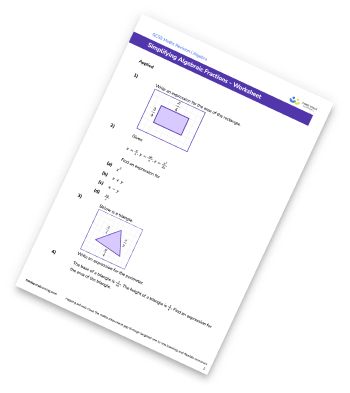Circle Graph Worksheet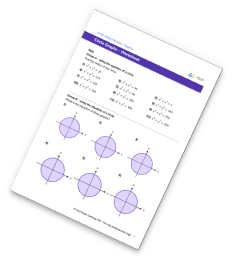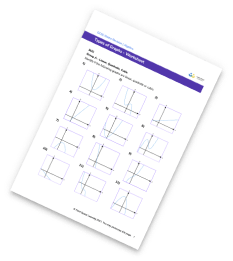Parallel And Perpendicular Lines Worksheet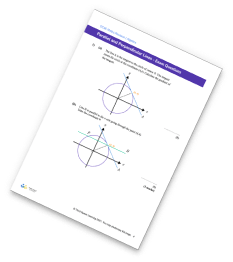## Do you have KS4 students who need more focused attention to succeed at GCSE?There will be students in your class who require individual attention to help them succeed in their maths GCSEs. In a class of 30, it’s not always easy to provide.

Help your students feel confident with exam-style questions and the strategies they’ll need to answer them correctly with our dedicated GCSE maths revision programme.

Lessons are selected to provide support where each student needs it most, and specially-trained GCSE maths tutors adapt the pitch and pace of each lesson. This ensures a personalised revision programme that raises grades and boosts confidence.

Find out more